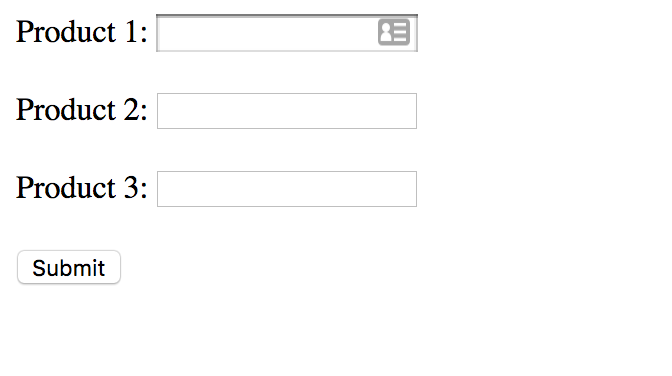# Validate an array input field

This tutorial is based on the procedural php. It will teach you how to validate an array input field with specific field. We find it really hard to validate an array input field. So this tutorial is make the concept more clear. The source code for this tutorial is available at end of the tutorial.

### Step 1: Setup the working environment

Go to the htdocs and create a folder named as validate_array_input and create a file index.php. Paste or write these code into the index.php

```<!DOCTYPE html>
<html>
<title>Validate the array input field form</title>
<body>

<form action="" method="post">

<?php for (\$i=1; \$i < 4; \$i++) { ?>
<div style="margin-bottom: 20px;">
<label>Product <?php echo \$i; ?>: </label>

<input type="text" name="productName[<?php echo \$i; ?>]" value="<?php echo \$_POST['productName'][\$i]; ?>"/>
</div>
<?php } // /.foreach ?>

<input type="submit" name="submitForm" value="Submit" />
</form>

</body>
</html>
```

As you can we can see we have create three input field by iterating for 3 times. So this means we will have three input filed.

```    for (\$i=1; \$i < 4; \$i++) {
}
```

This means it will iterate for 3 times, the \$i variable will repeat until its value is less than 4.

```    <input type="text" name="productName[<?php echo \$i; ?>]" value="<?php echo \$_POST['productName'][\$i]; ?>"/>
```

Now this input field is inside the iteration. This means the input field is repeated for three times with the array number 1, 2, and 3. This will represent as productName, productName, productName. The same logic goes to the value attribute of the input element.

If you have followed this steps correctly then the output of the code will look like below image:### Step 2: Work with php validation

We are almost finish with these tutorial, now we will add these codes as shown below.

```
\$errors = array();
if(isset(\$_POST['submitForm'])) {

\$productName = \$_POST['productName'];

foreach (\$productName as \$key => \$value) {
if(empty(\$productName[\$key])) {
\$errors[\$key] = "<p style='color:red;'> Product ".\$key." Field is Required</p> ";
}
} // /foreach

if(empty(\$errors)) {
// validation is ok, and here we can insert the data into the database
}

}
```

Add these code right above the html tag. This code checks if the form is submitted. If yes first it's stores the array data into the \$productName variable. After that it iterates through each array and checks if the value is empty or not. If it's empty then it's stores the error message into any error with the \$key variable which counts the array key number.

Another if statement checks if the \$errors variable is empty. If the \$errors is empty then the validation is validate.

```
if(!empty(\$errors)) {
foreach (\$errors as \$key => \$value) {
if(\$key == \$i) {
echo \$value;
} // .if
} // /.foreach errors
} // /.if
```

This code will be right below the input field to display the validation errors. The first if statement checks if the \$errors variable is not empty and if not empty then it iterates that variable to display that each message. As you know \$i variable is from for loop. Now it checks if the \$key and \$i variable matches with each other

### Integrate the step 2

By integrating the step 2 code it should look like below code

```
<?php

\$errors = array();
if(isset(\$_POST['submitForm'])) {

\$productName = \$_POST['productName'];

foreach (\$productName as \$key => \$value) {
if(empty(\$productName[\$key])) {
\$errors[\$key] = "<p style='color:red;'> Product ".\$key." Field is Required</p> ";
}
} // /foreach

if(empty(\$errors)) {
// validation is ok, and here we can insert the data into the database
}

}

?>

<!DOCTYPE html>
<html>
<title>Validate the array input field form</title>
<body>

<form action="" method="post">

<?php for (\$i=1; \$i < 4; \$i++) { ?>
<div style="margin-bottom: 20px;">
<label>Product <?php echo \$i; ?>: </label>

<input type="text" name="productName[<?php echo \$i; ?>]" value="<?php echo \$_POST['productName'][\$i]; ?>"/>
<?php
if(!empty(\$errors)) {
foreach (\$errors as \$key => \$value) {
if(\$key == \$i) {
echo \$value;
} // .if
} // /.foreach errors
} // /.if
?>
</div>
<?php } // /.foreach ?>

<input type="submit" name="submitForm" value="Submit" />
</form>

</body>
</html>
```

I hope this helps you alot.